NCERT Exemplar - MCQs

Chapter 5 Class 12 Continuity and Differentiability
Serial order wise

## (D) none of these.

This question is similar to Ex 5.1, 32 - Chapter 5 Class 12 and Ex 5.2, 9 - Chapter 5 Class 12 - Continuity and Differentiability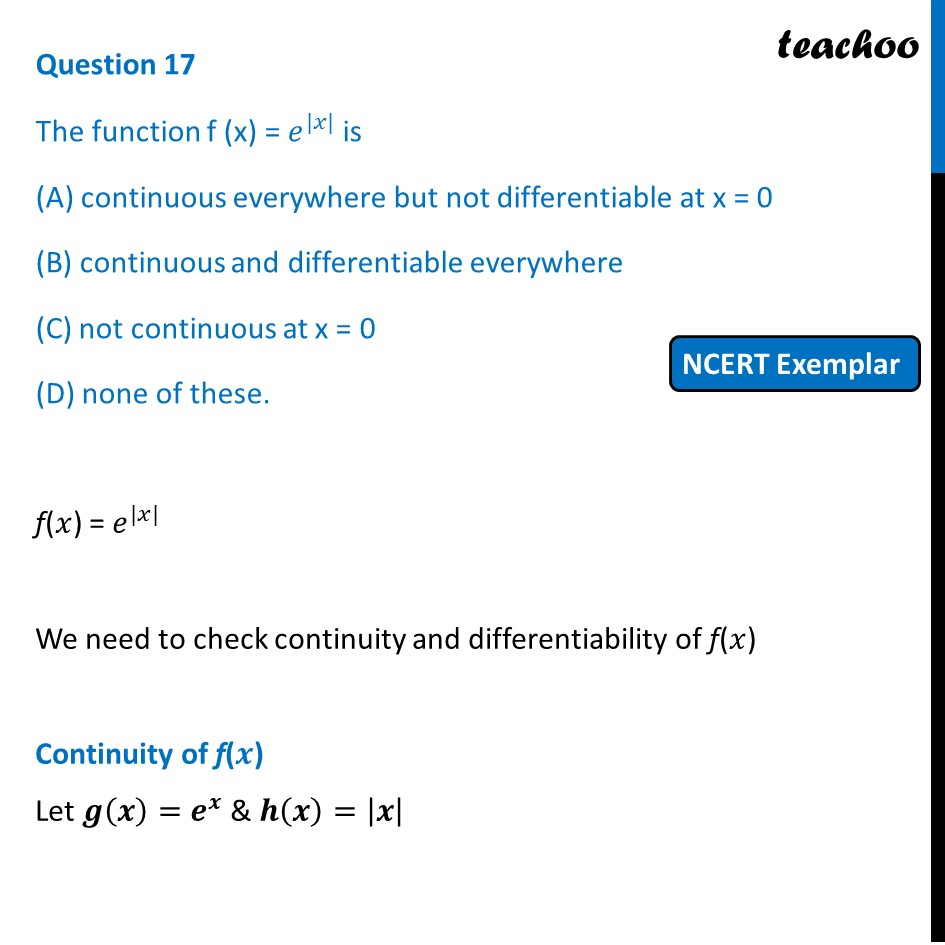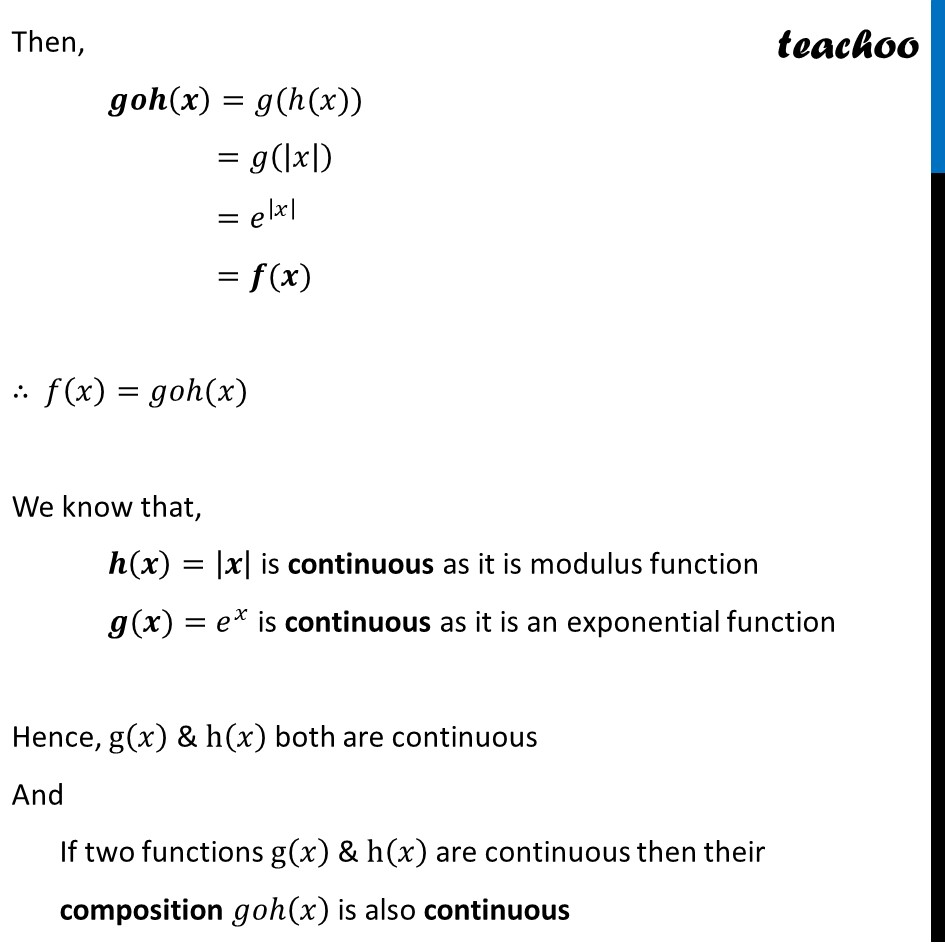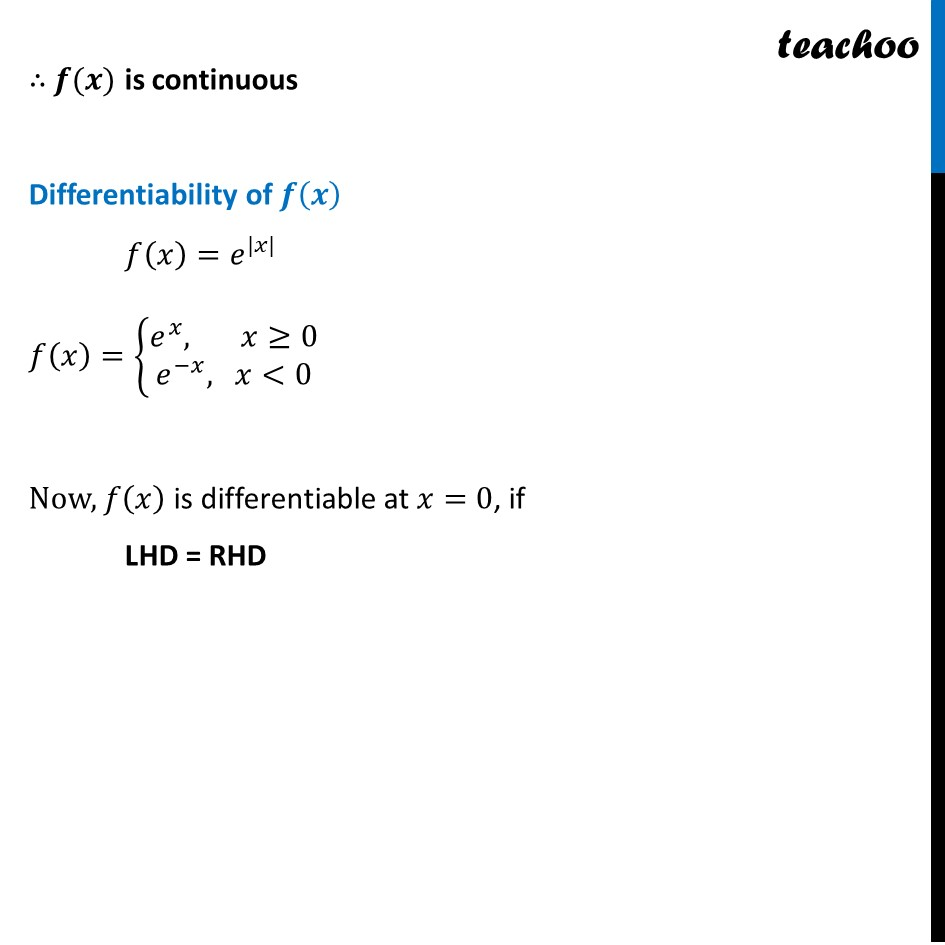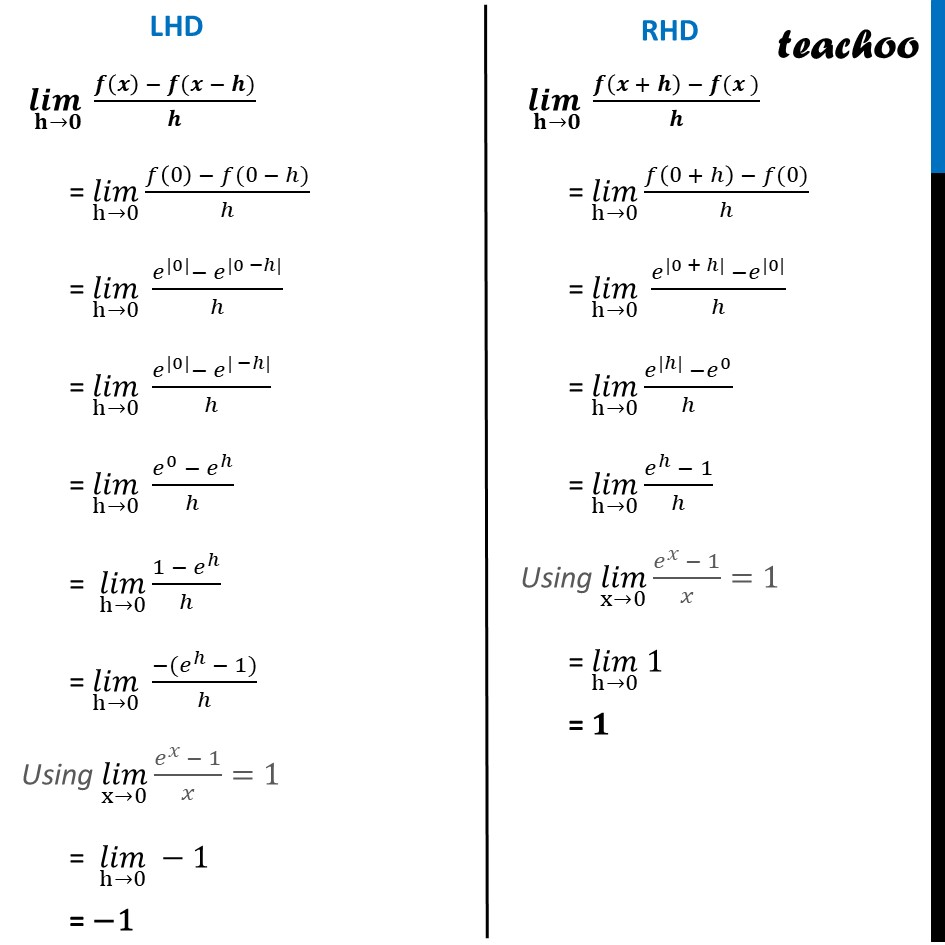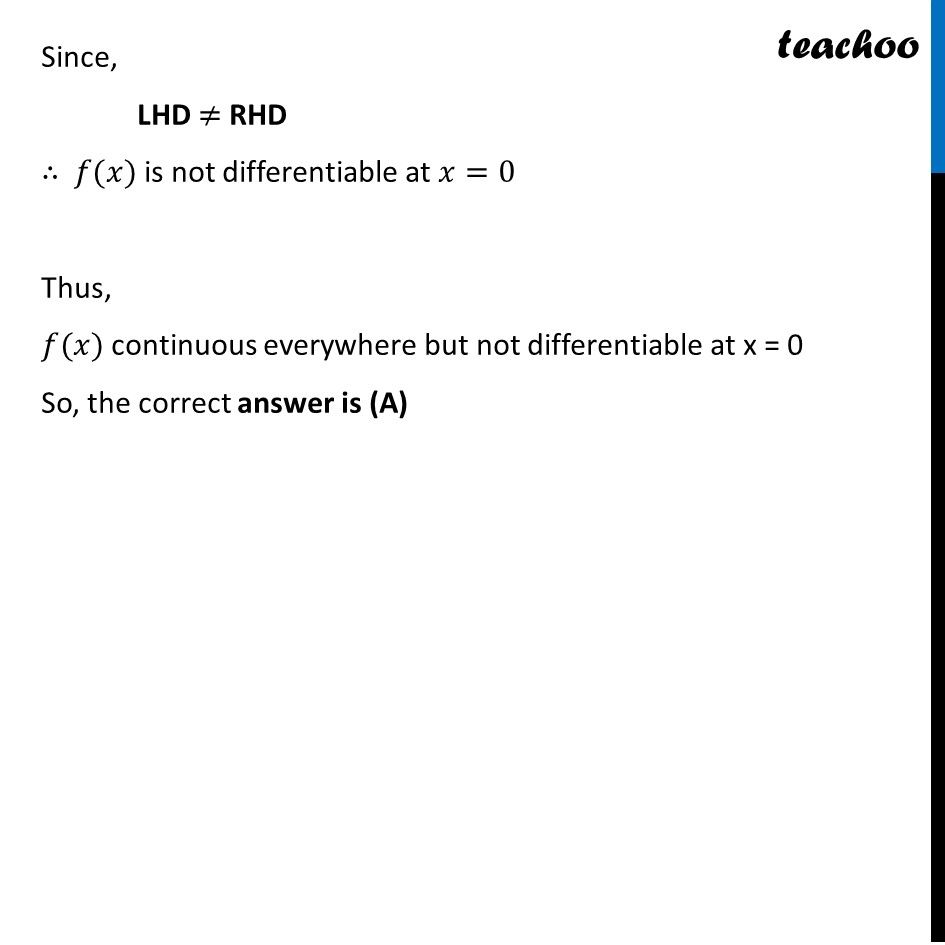Get live Maths 1-on-1 Classs - Class 6 to 12

### Transcript

Question 17 The function f (x) = 𝑒^(|𝑥|) is (A) continuous everywhere but not differentiable at x = 0 (B) continuous and differentiable everywhere (C) not continuous at x = 0 (D) none of these. f(𝑥) = 𝑒^(|𝑥|) We need to check continuity and differentiability of f(𝑥) Continuity of f(𝒙) Let 𝒈(𝒙)=𝒆^𝒙 & 𝒉(𝒙)=|𝒙| Then, 𝒈𝒐𝒉(𝒙)=𝑔(ℎ(𝑥)) =𝑔(|𝑥|) =𝑒^|𝑥| =𝒇(𝒙) ∴ 𝑓(𝑥)=𝑔𝑜ℎ(𝑥) We know that, 𝒉(𝒙)=|𝒙| is continuous as it is modulus function 𝒈(𝒙)=𝑒^𝑥 is continuous as it is an exponential function Hence, g(𝑥) & h(𝑥) both are continuous And If two functions g(𝑥) & h(𝑥) are continuous then their composition 𝑔𝑜ℎ(𝑥) is also continuous ∴ 𝒇(𝒙) is continuous Differentiability of 𝒇(𝒙) 𝑓(𝑥)=𝑒^(|𝑥|) 𝑓(𝑥)={■8(𝑒^𝑥, 𝑥≥[email protected]𝑒^(−𝑥), 𝑥<0)┤ Now, 𝑓(𝑥) is differentiable at 𝑥=0, if LHD = RHD (𝒍𝒊𝒎 )┬(𝐡→𝟎) (𝒇(𝒙) − 𝒇(𝒙 − 𝒉))/𝒉 = (𝑙𝑖𝑚)┬(h→0) (𝑓(0) − 𝑓(0 − ℎ))/ℎ = (𝑙𝑖𝑚)┬(h→0) (𝑒^(|0|)− 𝑒^(|0 −ℎ|))/ℎ = (𝑙𝑖𝑚)┬(h→0) (𝑒^(|0|)− 𝑒^(| −ℎ|))/ℎ = (𝑙𝑖𝑚)┬(h→0) (𝑒^0 − 𝑒^ℎ)/ℎ = (𝑙𝑖𝑚)┬(h→0) (1 − 𝑒^ℎ)/ℎ = (𝑙𝑖𝑚)┬(h→0) (−(𝑒^ℎ − 1))/ℎ Using (𝑙𝑖𝑚)┬(x→0) (𝑒^𝑥 − 1)/𝑥=1 = (𝑙𝑖𝑚)┬(h→0) −1 = −1 (𝒍𝒊𝒎 )┬(𝐡→𝟎) (𝒇(𝒙 + 𝒉) − 𝒇(𝒙 ))/𝒉 = (𝑙𝑖𝑚)┬(h→0) (𝑓(0 + ℎ) − 𝑓(0))/ℎ = (𝑙𝑖𝑚)┬(h→0) (𝑒^(|0 + ℎ|) −𝑒^(|0|))/ℎ = (𝑙𝑖𝑚)┬(h→0) (𝑒^(|ℎ|) −𝑒^0)/ℎ = (𝑙𝑖𝑚)┬(h→0) (𝑒^ℎ − 1)/ℎ Using (𝑙𝑖𝑚)┬(x→0) (𝑒^𝑥 − 1)/𝑥=1 = (𝑙𝑖𝑚)┬(h→0) 1 = 𝟏 Since, LHD ≠ RHD ∴ 𝑓(𝑥) is not differentiable at 𝑥=0 Thus, 𝑓(𝑥) continuous everywhere but not differentiable at x = 0 So, the correct answer is (A)